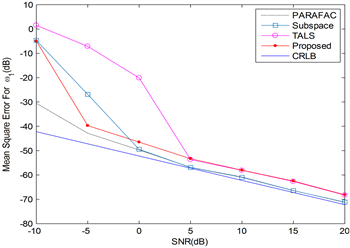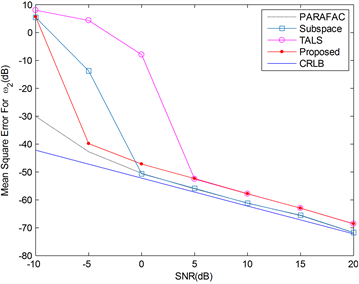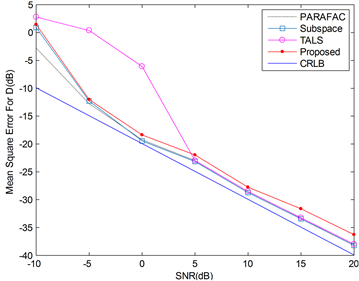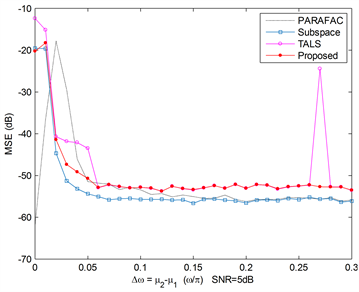# 基于Tucker分解的频率和时延联合估计算法Joint Frequency and Time-Delay Estimation Using Tucker Decomposition

DOI: 10.12677/JISP.2021.103011, PDF, HTML, XML, 下载: 25  浏览: 59  国家自然科学基金支持

Abstract: In this paper, we develop an algorithm based on tucker decomposition method for estimating the differential delay of a sinusoid signal received at two separated sensors as well as the sinusoidal frequencies. Using tucker method, the reconstructed signal tensor is decomposed, the frequency and time delay estimates are obtained by ESPRIT from the signal subspace. Performance evaluation via computer simulations is included to demonstrate the effectiveness of the proposed algorithm.

1. 引言

2. 问题描述及基本算法

$\begin{array}{l}{r}_{1}\left(n\right)=s\left(n\right)+{q}_{1}\left(n\right)\\ {r}_{2}\left(n\right)=s\left(n-D\right)+{q}_{2}\left(n\right),\text{}n=0,1,\cdots ,N-1\end{array}$ (1)

$x\left(n\right)={\left[{r}_{1}\left(n\right),{r}_{1}\left(n+1\right),\cdots ,{r}_{1}\left(n+M-1\right),{r}_{2}\left(n\right),{r}_{2}\left(n+1\right),\cdots ,{r}_{2}\left(n+M-1\right)\right]}^{\text{T}},\text{\hspace{0.17em}}n=0,1,\cdots ,K-1$ (2)

$s\left(n+1\right)=\Phi s\left( n \right)$

$x\left(n\right)=Bs\left(n\right)+q\left(n\right)$ (3)

$\text{A}=\left[\begin{array}{c}\text{1}\\ {\text{e}}^{j{\omega }_{1}}\\ \text{}⋮\\ {\text{e}}^{j{\omega }_{1}\left(M-1\right)}\end{array}\text{}\begin{array}{c}\text{1}\\ \begin{array}{l}{\text{e}}^{j{\omega }_{2}}\\ \text{}⋮\end{array}\\ {\text{e}}^{j{\omega }_{2}\left(M-1\right)}\end{array}\text{}\begin{array}{c}\cdots \\ \cdots \\ \ddots \\ \cdots \end{array}\text{}\begin{array}{c}\text{1}\\ \begin{array}{l}{\text{e}}^{j{\omega }_{p}}\\ \text{}⋮\end{array}\\ {\text{e}}^{j{\omega }_{p}\left(M-1\right)}\end{array}\right]$

$\Delta =\text{diag}\left({\text{e}}^{-jD{\omega }_{1}},{\text{e}}^{-jD{\omega }_{2}},\cdots ,{\text{e}}^{-jD{\omega }_{P}}\right)$

$q\left(n\right)={\left[{q}_{1}\left(n\right),{q}_{1}\left(n+1\right),\cdots ,{q}_{1}\left(n+M-1\right),{q}_{2}\left(n\right),{q}_{2}\left(n+1\right),\cdots ,{q}_{2}\left(n+M-1\right)\right]}^{\text{T}}$

$L\ge 2$ 是一给定的正整数，定义向量 ${X}_{l}=\left[x\left(1+l\right),\cdots ,x\left(K-L+l+1\right)\right]$，通过状态空间模型有：

${X}_{l}=B{\Phi }_{l}S+{Q}_{l},\text{}l=0,1,\cdots ,L-1$ (4)

$X\left(i,:,:\right)={x}_{n}\left(:,i:\left(i+L-1\right)\right),\text{\hspace{0.17em}}\text{\hspace{0.17em}}i=1,2,\cdots ,K-L+1$ (5)

$\mathcal{X}=\mathcal{G}×{}_{1}\text{U}{}_{1}×{}_{\text{2}}\text{U}{}_{\text{2}}×{}_{\text{3}}\text{U}{}_{3}$ (6)

$\stackrel{˜}{\mathcal{X}}={\stackrel{˜}{\mathcal{G}}}^{\left[P\right]}×{}_{1}\stackrel{˜}{U}{}_{1}^{\left[P\right]}×{}_{\text{2}}\stackrel{˜}{U}{}_{2}^{\left[P\right]}×{}_{\text{3}}\stackrel{˜}{U}{}_{3}^{\left[P\right]}$ (7)

$\stackrel{˜}{\Phi }={\left[{\stackrel{˜}{U}}_{1}^{\left[P\right]}\left(1:M-1\right),:\right]}^{\gamma }{\stackrel{˜}{U}}_{1}^{\left[P\right]}\left(P:M,:\right)$ (8)

$\stackrel{˜}{\Phi }={V}_{s}{\Lambda }_{s}{V}_{s}^{H}$ (9)

${\stackrel{˜}{\omega }}_{m}=\angle {\Lambda }_{s}\left(m,m\right),\text{}m=1,2,\cdots ,P$ (10)

$\stackrel{˜}{\Delta }={\left[{\stackrel{˜}{U}}_{2}^{\left[P\right]}\left(1:M,:\right)\right]}^{\gamma }{\stackrel{˜}{U}}_{2}^{\left[P\right]}\left(M+1:2M,:\right)$ (11)

$\stackrel{^}{D}=\frac{{\sum }_{m=1}^{P}\angle \stackrel{˜}{\Delta }\left(m,m\right)}{-{\sum }_{m=1}^{P}{\stackrel{^}{\omega }}_{m}}$ (12)

3. 仿真结果Figure 1. MSE versus SNR for Frequency ${\omega }_{1}$Figure 2. MSE versus SNR for Frequency ${\omega }_{2}$Figure 3. MSE versus SNR for Frequency ${\omega }_{3}$Figure 4. Frequency resolutionFigure 5. Computational time compared

4. 结论

  Sherman, D.L., Tsai, Y.C., Rossell, L.A., et al. (1995) Narrowband Delay Estimation for Thalamocortical Epileptic Seizure Pathways. 1995 International Conference on Acoustics, Speech, and Signal Processing IEEE, Vol. 5, 2939-2942.  Sills, J.A. and Black, Q.R. (1996) Frequency Estimation from Short Pulses of Sinusoidal Signals. Proceedings of MILCOM’96 IEEE Military Communications Conference IEEE, Vol. 3, 979-983.  Ngan, L.Y., Wu, Y., So, H.C., et al. (2003) Joint Time Delay and Pitch Estimation for Speaker Localization. Proceedings of the 2003 International Symposium on Circuits and Systems, Vol. 3, 3.  Wu, Y., So, H.C. and Tan, Y. (2009) Joint Time-Delay and Frequency Estimation Using Parallel Factor Analysis. Signal Processing, 89, 1667-1670. https://doi.org/10.1016/j.sigpro.2009.03.004  Liao, G., So, H.C. and Ching, P.C. (2001) Joint Time Delay and Frequency Estimation of Multiple Sinusoids. 2001 IEEE International Conference on Acoustics, Speech, and Signal Processing, Vol. 5, 3121-3124.  Wu, Y., So, H.C. and Ching, P.C. (2003) Joint Time Delay and Frequency Estimation via State-Space Realization. IEEE Signal Processing Letters, 10, 339-342. https://doi.org/10.1109/LSP.2003.817854  Zhang, X.F. and Xu, D.Z. (2009) Novel Joint Time Delay and Frequency Estimation Method. IET Radar, Sonar & Navigation, 3, 186-194. https://doi.org/10.1049/iet-rsn:20080032  Stoica, P. and Nehorai, A. (1989) MUSIC, Maximum Likelihood, and Cramer-Rao Bound. IEEE Transactions on Acoustics, Speech, and Signal Processing, 37, 720-741. https://doi.org/10.1109/29.17564  Tucker, L.R. (1963) Implications of Factor Analysis of Three-Way Matrices for Measurement of Change. Problems in Measuring Change, 15, 122-137.  Tucker, L.R. (1966) Some Mathematical Notes on Three-Mode Factor Analysis. Psychometrika, 31, 279-311. https://doi.org/10.1007/BF02289464  Kapteyn, A., Neudecker, H. and Wansbeek, T. (1986) An Approach Ton-Mode Components Analysis. Psychometrika, 51, 269-275. https://doi.org/10.1007/BF02293984  De Lathauwer, L., De Moor, B. and Vandewalle, J. (2000) A Multilinear Singular Value Decomposition. SIAM Journal on Matrix Analysis and Applications, 21, 1253-1278. https://doi.org/10.1137/S0895479896305696  Van Trees, H.L. (2004) Optimum Array Processing: Part IV of Detection, Estimation, and Modulation Theory. John Wiley & Sons, Hoboken.  吴云韬. 非平稳, 色噪声环境下的参数估计方法研究[D]: [博士学位论文]. 西安: 西安电子科技大学, 2003.  Kolda, T.G. and Bader, B.W. (2009) Tensor Decompositions and Applications. SIAM Review, 51, 455-500. https://doi.org/10.1137/07070111X  Roy, R. and Kailath, T. (1989) ESPRIT-Estimation of Signal Parameters via Rotational Invariance Techniques. IEEE Transactions on Acoustics, Speech, and Signal Processing, 37, 984-995. https://doi.org/10.1109/29.32276  Bro, R.P. (1997) Tutorial and Applications. Chemometrics and Intelligent Laboratory Systems, 38, 149-172.  张胜男. 基于平行因子分析的阵列参数估计[D]: [硕士学位论文]. 南京: 南京航空航天大学, 2008.